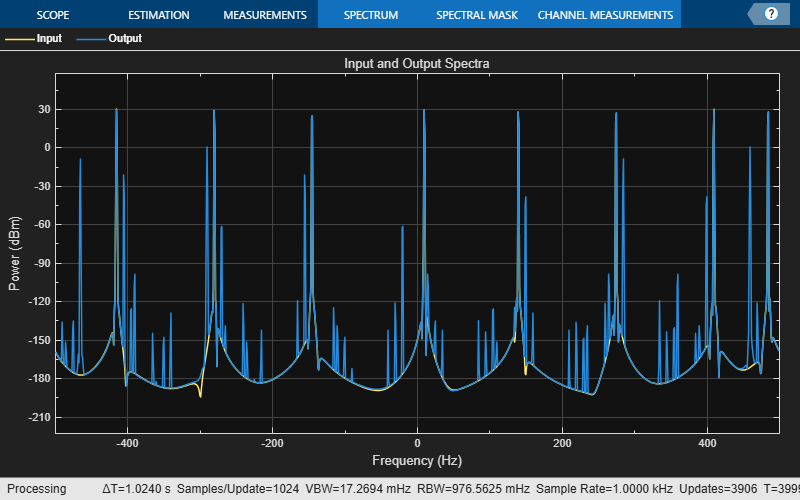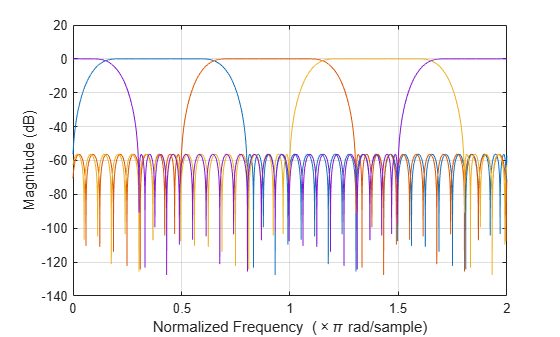# dsp.Channelizer

Polyphase FFT analysis filter bank

## Description

The `dsp.Channelizer` System object™ separates a broadband input signal into multiple narrow subbands using a fast Fourier transform (FFT)-based analysis filter bank. The filter bank uses a prototype lowpass filter and is implemented using a polyphase structure. You can specify the filter coefficients directly or through design parameters.

To separate a broadband signal into multiple narrow subbands:

1. Create the `dsp.Channelizer` object and set its properties.

2. Call the object with arguments, as if it were a function.

## Creation

### Syntax

``channelizer = dsp.Channelizer``
``channelizer = dsp.Channelizer(M)``
``channelizer = dsp.Channelizer(M,D)``
``channelizer = dsp.Channelizer(Name,Value)``

### Description

example

````channelizer = dsp.Channelizer` creates a polyphase FFT analysis filter bank System object that separates a broadband input signal into multiple narrowband output signals. This object implements the inverse operation of the `dsp.ChannelSynthesizer` System object.```

example

````channelizer = dsp.Channelizer(M)` creates an M-band polyphase FFT analysis filter bank, with the `NumFrequencyBands` property set to M. Example: `channelizer = dsp.Channelizer(16)````
````channelizer = dsp.Channelizer(M,D)` creates an M-band polyphase FFT analysis filter bank, with the `DecimationFactor` property set to D. Example: channelizer = dsp.Channelizer(16,8);```

example

````channelizer = dsp.Channelizer(Name,Value)` creates a polyphase FFT analysis filter bank with each specified property set to the specified value. Enclose each property name in single quotes. For example, you can set `NumTapsPerBand` to 20 and `StopbandAttenuation` to 140.```

## Properties

expand all

Unless otherwise indicated, properties are nontunable, which means you cannot change their values after calling the object. Objects lock when you call them, and the `release` function unlocks them.

If a property is tunable, you can change its value at any time.

### Main Properties

Number of frequency bands M into which the object separates the input broadband signal, specified as a positive integer greater than 1. This property corresponds to the number of polyphase branches and the FFT length used in the filter bank.

Example: 16

Example: 64

Data Types: `single` | `double` | `int8` | `int16` | `int32` | `int64` | `uint8` | `uint16` | `uint32` | `uint64`

Decimation factor D specified as a positive integer less than or equal to the number of frequency bands M. The default value of this property equals the number of frequency bands specified.

If the decimation factor D equals the number of frequency bands M, then the M/D ratio equals 1, and the channelizer is known as the maximally decimated channelizer.

If the M/D ratio is greater than `1`, the output sample rate is different from the channel spacing, and the channelizer is known as the non-maximally decimated channelizer. If the ratio is an integer, the channelizer is known as the integer-oversampled channelizer. If the ratio is not an integer, say 4/3, the channelizer is known as the rationally oversampled channelizer. For more details, see Algorithm.

Data Types: `single` | `double` | `int8` | `int16` | `int32` | `int64` | `uint8` | `uint16` | `uint32` | `uint64`

Filter design parameters or filter coefficients, specified as one of these options:

Number of filter coefficients each polyphase branch uses, specified as a positive integer. The number of polyphase branches matches the number of frequency bands. The total number of filter coefficients for the prototype lowpass filter is given by `NumFrequencyBands` × `NumTapsPerBand`. For a given stopband attenuation, increasing the number of taps per band narrows the transition width of the filter. As a result, there is more usable bandwidth for each frequency band at the expense of increased computation.

Example: 8

Example: 16

#### Dependencies

This property applies when you set `Specification` to `"Number of taps per band and stopband attenuation"`.

Data Types: `single` | `double` | `int8` | `int16` | `int32` | `int64` | `uint8` | `uint16` | `uint32` | `uint64`

Stopband attenuation of the lowpass filter, specified as a positive real scalar in dB. This value controls the maximum amount of aliasing from one frequency band to the next. When the stopband attenuation increases, the passband ripple decreases. For a given stopband attenuation, increasing the number of taps per band narrows the transition width of the filter. As a result, there is more usable bandwidth for each frequency band at the expense of increased computation.

Example: 80

#### Dependencies

This property applies when you set `Specification` to `"Number of taps per band and stopband attenuation"`.

Data Types: `single` | `double`

Coefficients of the prototype lowpass filter, specified as a row vector. The default vector of coefficients is obtained using `rcosdesign(0.25,6,8,"sqrt")`. There must be at least one coefficient per frequency band. If the length of the lowpass filter is less than the number of frequency bands, the object zero-pads the coefficients.

If you specify complex coefficients, the object designs a prototype filter that is centered at a nonzero frequency, also known as a bandpass filter. The modulated versions of the prototype bandpass filter appear with respect to the prototype filter and are wrapped around the frequency range [−Fs Fs]. For an example, see Channelizer with Complex Coefficients.

Tunable: Yes

#### Dependencies

This property applies when you set `Specification` to `"Coefficients"`.

Data Types: `single` | `double`
Complex Number Support: Yes

### Code Generation Properties

Allow arbitrary frame length for fixed-size input signals in the generated code, specified as `true` or `false`.

When you specify:

• `true` –– The input frame length does not have to be a multiple of the decimation factor. The output of the object in the generated code is a variable-size array.

• `false` –– The input frame length must be a multiple of the decimation factor.

When you specify variable-size signals, the input frame length can be arbitrary and the object ignores this property in the generated code. When you run this object in MATLAB®, the object supports arbitrary input frame lengths for fixed-size and variable-size signals and this property does not affect the object behavior.

Data Types: `logical`

## Usage

### Syntax

``channOut = channelizer(input)``

### Description

example

````channOut = channelizer(input)` separates the broadband input signal into a number of narrow band signals contained in the columns of the channelizer output. ```

### Input Arguments

expand all

Data input, specified as an L-by-1 vector or an L-by-N matrix, with N > 1.

Under most conditions, the number of inputs rows L can be arbitrary and does not have to be a multiple of the number of frequency bands. See this table for details.

Input SignalWhen you Run Object in MATLABWhen you Generate Code Using MATLAB Coder™
Fixed-sizeObject supports arbitrary input frame lengthObject supports arbitrary input frame length when you set `AllowArbitraryInputLength` to `true` while generating code
Variable-sizeObject supports arbitrary input frame lengthObject supports arbitrary input frame length

Variable-size signals change in frame length once you lock the object while the fixed-size signals remain constant. When the object does not support arbitrary frame length, the input frame length must be a multiple of the number of frequency bands.

Data Types: `single` | `double`
Complex Number Support: Yes

### Output Arguments

expand all

Channelizer output, returned as a matrix or a 3-D array.

If the input is an L-by-1 vector, then the output signal has an upper bound size of `ceil`(L/M)-by-M, where M is the number of frequency bands. Each narrowband signal forms a column in the output.

If the input has more than one channel, that is, it is of the form L-by-N with N > 1, then the output signal has an upper bound size of `ceil`(L/M)-by-M-by-N.

Data Types: `single` | `double`
Complex Number Support: Yes

## Object Functions

To use an object function, specify the System object as the first input argument. For example, to release system resources of a System object named `obj`, use this syntax:

`release(obj)`

expand all

 `coeffs` Coefficients of prototype lowpass filter `tf` Return transfer function of overall prototype lowpass filter `polyphase` Return polyphase matrix `freqz` Frequency response of filters in channelizer `fvtool` Visualize the filters in the channelizer `bandedgeFrequencies` Compute the bandedge frequencies `centerFrequencies` Compute center frequencies `getFilters` Return matrix of channelizer FIR filters
 `step` Run System object algorithm `release` Release resources and allow changes to System object property values and input characteristics `reset` Reset internal states of System object

## Examples

collapse all

Channelize and synthesize a sine wave signal with multiple frequencies using an M -channel filter bank.

The M -channel filter bank contains an analysis filter bank section and a synthesis filter bank section. The `dsp.Channelizer` object implements the analysis filter bank section. The `dsp.ChannelSynthesizer` object implements the synthesis filter bank section. These objects use an efficient polyphase structure to implement the filter bank. For more details, see Polyphase Implementation under Algorithms on the object reference pages.

Initialization

Initialize the `dsp.Channelizer` and `dsp.ChannelSynthesizer` System objects. Each object is set up with 8 frequency bands, 8 polyphase branches in each filter, 12 coefficients per polyphase branch, and a stopband attenuation of 140 dB. Use a sine wave with multiple frequencies as the input signal. View the input spectrum and the output spectrum using a spectrum analyzer.

```offsets = [-40,-30,-20,10,15,25,35,-15]; sinewave = dsp.SineWave('ComplexOutput',true,'Frequency',... offsets+(-375:125:500),'SamplesPerFrame',800); channelizer = dsp.Channelizer('StopbandAttenuation',140); synthesizer = dsp.ChannelSynthesizer('StopbandAttenuation',140); spectrumAnalyzer = spectrumAnalyzer('ShowLegend',true,... 'SampleRate',sinewave.SampleRate,... 'ChannelNames',{'Input','Output'},... 'Title',"Input and Output Spectra");```

Streaming

Use the channelizer to split the broadband input signal into multiple narrow bands. Then pass the multiple narrowband signals into the synthesizer, which merges these signals to form the broadband signal. Compare the spectra of the input and output signals. The input and output spectra match very closely.

```for i = 1:5000 x = sum(sinewave(),2); y = channelizer(x); v = synthesizer(y); spectrumAnalyzer(x,v) end```Create a `dsp.Channelizer` object and set the `LowpassCoefficients `property to a vector of complex coefficients.

Complex Coefficients

Using `firpm`, determine the coefficients of a Park-McClellan's optimal equiripple FIR filter of order 30, and frequency and amplitude characteristics described by F = [0 0.2 0.4 1.0] and A = [1 1 0 0] vectors, respectively.

Create a complex version of these coefficients by multiplying with a complex exponential. The resultant frequency response is that of a bandpass filter at the specified frequency, in this case 0.4.

```blowpass = firpm(30,[0 .2 .4 1],[1 1 0 0]); N = length(blowpass)-1; Fc = 0.4; j = complex(0,1); bbandpass = blowpass.*exp(j*Fc*pi*(0:N));```

Channelizer

Create a `dsp.Channelizer` object with 4 frequency bands and set the `Specification` property to `'Coefficients'`.

`chann = dsp.Channelizer(4,'Specification',"Coefficients");`

Pass the complex coefficients to the channelizer. The prototype filter is a bandpass filter with a center frequency of 0.4. The modulated versions of this filter appear with respect to the prototype filter and are wrapped around the frequency range [$-$Fs Fs].

`chann.LowpassCoefficients = bbandpass`
```chann = dsp.Channelizer with properties: Main NumFrequencyBands: 4 DecimationFactor: 4 Specification: 'Coefficients' LowpassCoefficients: [0.0019 + 0.0000i 0.0005 + 0.0016i 0.0011 - 0.0008i 0.0051 + 0.0037i -0.0024 + 0.0073i -3.4175e-04 + 8.3704e-20i 0.0042 + 0.0128i -0.0174 + 0.0126i -0.0077 - 0.0056i -0.0070 + 0.0214i -0.0514 + 0.0000i -0.0127 - 0.0392i ... ] Show all properties ```

Visualize the frequency response of the channelizer.

`fvtool(chann)`